Impact Factor 3.560 | CiteScore 3.1
More on impact ›

# Frontiers in Physics## ORIGINAL RESEARCH article

Front. Phys., 30 May 2019 | https://doi.org/10.3389/fphy.2019.00071

# Determination of the Effective Viscosity of Non-newtonian Fluids Flowing Through Porous MediaUrsin Eberhard1*,Hansjoerg J. Seybold1,Marius Floriancic1,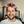Pascal Bertsch1,Joaquin Jiménez-Martínez1,2,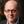José S. Andrade Jr.3 and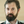Markus Holzner1
• 1ETH Zürich, Zurich, Switzerland
• 2Eawag, Dübendorf, Switzerland
• 3Departamento de Física, Universidade Federal do Ceara, Fortaleza, Brazil

When non-Newtonian fluids flow through porous media, the topology of the pore space leads to a broad range of flow velocities and shear rates. Consequently, the local viscosity of the fluid also varies in space with a non-linear dependence on the Darcy velocity. Therefore, an effective viscosity μeff is usually used to describe the flow at the Darcy scale. For most non-Newtonian flows the rheology of the fluid can be described by a (non linear) function of the shear rate. Current approaches estimate the effective viscosity by first calculating an effective shear rate mainly by adopting a power-law model for the rheology and including an empirical correction factor. In a second step this averaged shear rate is used together with the real rheology of the fluid to calculate μeff. In this work, we derive a semi-analytical expression for the local viscosity profile using a Carreau type fluid, which is a more broadly applicable model than the power-law model. By solving the flow equations in a circular cross section of a capillary we are able to calculate the average viscous resistance 〈μ〉 directly as a spatial average of the local viscosity. This approach circumvents the use of classical capillary bundle models and allows to upscale the viscosity distribution in a pore with a mean pore size to the Darcy scale. Different from commonly used capillary bundle models, the presented approach does neither require tortuosity nor permeability as input parameters. Consequently, our model only uses the characteristic length scale of the porous media and does not require empirical coefficients. The comparison of the proposed model with flow cell experiments conducted in a packed bed of monodisperse spherical beads shows, that our approach performs well by only using the physical rheology of the fluid, the porosity and the estimated mean pore size, without the need to determine an effective shear rate. The good agreement of our model with flow experiments and existing models suggests that the mean viscosity 〈μ〉 is a good estimate for the effective Darcy viscosity μeff providing physical insight into upscaling of non-Newtonian flows in porous media.

## 1. Introduction

Flow through porous media is ubiquitous in many natural and industrial systems. Examples include flow through biological tissues, blood vessels and bones  or through soils, sediments and rocks, with long-standing interest in hydrology [4, 5], petroleum , and chemical engineering . At low Reynolds numbers (Re≪1) the bulk flow of a Newtonian fluid flowing through porous media is described by Darcy's law

where q is the mean flow rate per unit area, also called Darcy velocity and μ is the dynamic viscosity. The variable κ is the permeability and Δp/L is the pressure drop over the distance L. The proportionality constant K = κ/μ is called hydraulic conductivity and can be derived from Stokes' equation assuming a linear relation between the viscous forces and the flow velocity .

While Darcy's law is a sound description for the bulk behavior of a fluid whose viscosity μ is constant, many relevant fluids in e.g., in food  and petroleum [14, 15] industry, show a much more complex constitutive law. For most of these so-called non-Newtonian fluids, the viscosity can be described by a nonlinear function of the stress-strain rate tensor E or more specifically its first principal invariant $\stackrel{˙}{\gamma }=\frac{1}{2}\sqrt{\text{E}:\text{E}}$ . Due to the heterogeneity of the flow velocities in the interstitial pore space, shear rates vary considerably inside the porous media. For non-Newtonian flows the coupling of the constitutive equations with the flow field leads to a spatial variable viscous resistance. Consequently, the relation between Darcy velocity and pressure drop cannot be described by a linear function anymore as in the case of Newtonian fluids. In order to obtain a bulk equation for the flow that is linear in the pressure drop, an effective viscosity μeff—which itself depends on the flow variables—must be used in order to account for the non-linear effects i.e.,

Here we assumed that the permeability κ is a characteristic constant representing the complexity of the pore space alone. Several empirical and semi-empirical models have been proposed to estimate μeff . Most of these models start from a capillary bundle representation of the different flow paths through a porous medium and estimate an effective shear rate ${\stackrel{˙}{\gamma }}_{\text{eff}}$ by comparing the flow rate of a power-law fluid with that of a Newtonian Poiseuille flow  (see also SI). Although analytical solutions can be derived to determine ${\stackrel{˙}{\gamma }}_{\text{eff}}$ for power-law rheologies, previous studies proposed various empirical correction factors [19, 20] to relate Darcy velocity to the effective shear rate. The effective shear rate ${\stackrel{˙}{\gamma }}_{\text{eff}}$ is then inserted into the constitutive law of the fluid of interest $\mu \left(\stackrel{˙}{\gamma }\right)$ to obtain an effective viscosity μeff. This approach requires an empirical factor to relate q to ${\stackrel{˙}{\gamma }}_{\text{eff}}$, which can vary over several orders of magnitude [25, 26], depending on the properties of the fluid, the tortuosity and the permeability. This suggests that the above assumptions are questionable. Additionally most of these models predict a linear relationship between the effective shear rate and the Darcy velocity.

In this manuscript we show that for a Carreau fluid , the local viscosity can be derived directly from the fluid's constitutive law and the velocity profile in a mean pore size, using a circular capillary to mimic the flow at pore scale. Contrary to commonly used capillary bundle models, our approach does neither require the knowledge of the tortuosity nor of the permeability. The capillary is only used to calculate a fully developed average flow profile. Finally, we calculate the mean viscous resistance by spatially averaging the local viscosity 〈μ〉. Comparisons of our results with flow cell experiments and existing models show that 〈μ〉 is a good estimate for μeff.

## 2. Methodology

### 2.1. Characterization of the Fluid

In order to model the flow of a non-Newtonian fluid, we first need to characterize its constitutive behavior. For most non-Newtonian fluids the constitutive relation between the deviatoric stress tensor T and the applied strain rates E can be described by a time independent scalar function $\mu =\mu \left(\stackrel{˙}{\gamma }\right)$, such that $T=2\mu \left(\stackrel{˙}{\gamma }\right)\text{E}$. Here μ is a generalized viscosity which depends only on the first principal invariant $\stackrel{˙}{\gamma }=\frac{1}{2}\sqrt{\text{E}:\text{E}}$ of the stress-strain rate tensor E . In the case of simple shear flow $\stackrel{˙}{\gamma }$ reduces to the shear rate. Many functional forms for $\mu \left(\stackrel{˙}{\gamma }\right)$ have been proposed, where the most common ones are the power-law model (Figure 1A), the Carreau model (Figure 1B), the Cross model or the Herschel-Bulkley model [18, 28].

FIGURE 1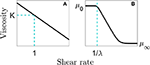Figure 1. Sketch of two shear thinning rheologies: (A) pure power-law model with two parameters K and n, (B) Carreau model with five parameters μ0, μ, λ, n and α.

The power-law model is described by

where K is the viscosity at the shear rate $\stackrel{˙}{\gamma }=1$ s−1 and n is the power-law index defining the steepness of the shear-thinning decay for n < 1 (see Figure 1). Due to its simplicity, the power-law model is the most commonly used rheology to derive analytical expressions. However, the unbounded power-law model has two drawbacks: first the model does not capture the linear shear-strain relation for very low and very high shear rates, that are prevalent in most natural systems, and second the viscosity curve becomes singular in the limit of vanishing shear. Consequently constitutive models which “blend” a power-law regime between a Newtonian behavior at low and high shear rates—such as the Carreau model—have been proposed for real world applications. The constitutive law of a Carreau fluid is parametrized by

where n is the power-law exponent, μ0 and μ are the limits of the viscosity at zero and infinite shear and λ is the reciprocal of the critical shear rate, which describes the onset of the shear thinning regime. The parameter α describes how smoothly the Newtonian regime blends into the power-law.

### 2.2. Current Models

Most commonly applied models to estimate μeff can be derived by equating the flow rate of a Poiseuille flow  with the flow rate of a power-law fluid 

For a circular capillary with radius R one obtains

where we used

which is also known as the Rabinowitsch equation  to describe the flow rate of a power-law fluid in a capillary. Solving Equation (6) for μ, a power-law viscosity μpower−law can be defined as

Equation (8) can be simplified to

This power-law viscosity corresponds to the viscosity of a Newtonian fluid which would have given the same pressure drop Δp/L along a capillary.

For a power-law constitutive relation $\mu =K{\stackrel{˙}{\gamma }}^{n-1}$ Equation (9) can be inverted to obtain an effective shear rate ${\stackrel{˙}{\gamma }}_{\text{eff}}$

Using the Rabinowitsch equation, we can express the term ${\left(\frac{\Delta p}{KL}\right)}^{\frac{1}{n}}$ as

where qcap is the mean capillary velocity defined as ${Q}_{\text{power}-\text{law}}/\left(\pi {R}^{2}\right)$. Furthermore, the mean capillary velocity qcap can be defined as the Darcy velocity divided by the porosity, ${q}_{\text{cap}}=\frac{q}{\Phi }$. Following Savins , the radius Req can be expressed by

where ζ is the tortuosity, Req is the radius of a capillary in the capillary bundle model (see detailed derivation in Supplementary Information). Inserting Req into Equation (10) then yields

Empirically it has been found by Cannella et al.  that the factor $1/\sqrt{\zeta }$ does not fit realistic data and replaced the term $1/\sqrt{\zeta }$ by a constant C, i.e.,

Hirasaki and Pope  proposed to use $C=1/\sqrt{25/12}\approx 0.69$ by using the tortuosity ζ of packed spheres, which has been widely reported to be 25/12 [32, 33]. Ignoring the tortuosity ζ, Cannella et al.  found a factor of C = 6 to be suitable to describe many flows in different settings. Additionally, Cannella et al. accounted for unsaturated and multiphase flows by correcting the permeability κ to κr,wκ and the porosity Φ to SwΦ. Here, κr,w is the relative permeability and Sw is the saturation. Consequently, the effective shear rate according to Cannella et al. [19, 26] is given by

Cannella et al. then used this effective shear rate together with a constitutive law $\mu \left(\stackrel{˙}{\gamma }\right)$ to calculate an effective viscosity. For this last step, mostly the Carreau model has been used due to its ability to fit a wide range of different rheologies.

Other models, that have been developed, are using more complex rheological descriptions of the fluid. Nevertheless, they generally require to correct the analytical solution with empirical factors to achieve reasonable agreement with experimental data .

### 2.3. Average Viscosity Approach

Here we present a new approach to estimate μeff by solving directly for the viscosity profile of a fully developed Carreau flow inside a single capillary of radius R that mimics a mean pore with a mean flow rate of $\frac{q}{\Phi }$. This approach assumes that a single constitutive law can be used both on the pore as well as on Darcy scale. This assumption is supported by the observation that even at pore scale, the viscosity distribution covers the whole range of viscosities given by the Carreau model equation. Consequently a single power-law is insufficient to describe the transitional behavior at the low and high shear limits.The onset of the power-law regime occurs at a characteristic combination of the Darcy velocity and the pore size. Therefore, it is important to determine at which Darcy velocity the onset of the non-linearities of the fluid starts to matter for a given pore size.

The Carreau model allows to obtain an average viscosity profile in a pore without invoking an effective shear rate and an intermediate power-law rheology. Note that the Carreau model includes the critical shear rate 1/λ, which defines the onset of the power-law regime, see Figure 1. To model the flow of a Carreau fluid we perform the following steps:

(i) We estimate the characteristic pore size in order to set the diameter of the average pore for which we examine the flow profile. This characteristic pore size can be readily obtained from an pore- or grain-size distribution. (ii) We then compute the velocity profile as a function of the flow rate. (iii) The velocity profile obtained in the previous step can subsequently be used to determine local shear rates $\stackrel{˙}{\gamma }\left(r\right)=\text{d}u\left(r\right)/\text{d}r$. (iv) Combining the shear and the Carreau rheology (Equation 4) we obtain the local viscosity distribution $\mu \left(\stackrel{˙}{\gamma }\left(r\right)\right)$ in a cross section of the capillary. (v) Finally, we use the local viscosity, to estimate the effective viscosity μeff by averaging the viscosity profile over the cross section of the capillary.

In order to apply this concept of a mean profile in a pore, we use a capillary with a circular cross section and assume a fully developed flow profile. The steady state Navier-Stokes equation at low Reynolds numbers in a circular capillary can be written as

where the pressure gradient along the capillary is constant $\frac{\text{d}p}{\text{d}x}=const$. Integrating with respect to r yields

Based on the symmetry of the flow profile, the velocity is maximal along the center line of the capillary at r = 0. By definition of a maximum, the shear rate $\stackrel{˙}{\gamma }=\frac{\text{d}u}{\text{d}r}$ vanishes, $\frac{\text{d}u}{\text{d}r}{|}_{r=0}=0$, which results in K1 = 0. Thus, Equation (17) simplifies to

Since the pressure drop $\frac{\text{d}p}{\text{d}x}$ along the capillary is assumed to be constant, we can replace it by a reference pressure pref ≠ 0 divided by a reference length scale. Choosing the capillary radius R as characteristic length, we define

Then, we insert the reference pressure from Equation (19) into Equation (18) and obtain

Inserting the constitutive law of a Carreau fluid (Equation 4) into (Equation 20) and solving for r yields

This expression can be rewritten by using the boundary condition for the shear rate ($\stackrel{˙}{\gamma }{|}_{r=R}=-{\stackrel{˙}{\gamma }}_{w}$), where ${\stackrel{˙}{\gamma }}_{w}$ is the shear rate at the wall of the capillary. Consequently, the reference pressure is given by the following equation:

In order to obtain an expression for the flow profile u(r) and ${\stackrel{˙}{\gamma }}_{w}$, we integrate $r\left({\stackrel{˙}{\gamma }}^{\prime }\right)$ given by Equation (21) radially with respect to ${\stackrel{˙}{\gamma }}^{\prime }$. As the shear rate ${\stackrel{˙}{\gamma }}^{\prime }\left(r\right)$ is an odd function, the relation ${\int }_{0}^{-\stackrel{˙}{\gamma }}\text{d}{\stackrel{˙}{\gamma }}^{\prime }=-{\int }_{0}^{\stackrel{˙}{\gamma }}\text{d}{\stackrel{˙}{\gamma }}^{\prime }$ holds for all r. Consequently, the shear rate $\stackrel{˙}{\gamma }$ will be our free parameter ranging from 0 to $-{\stackrel{˙}{\gamma }}_{w}$.

The resulting integral can be expressed as

Here 2F1(a, b; c; z) is a hypergeometric function with parameters a, b and c. Further details about the hypergeometric function can be found in the Handbook of mathematical functions by M. Abramowitz and I.A. Stegun . Using the chain rule on $\text{d}\left(r{\stackrel{˙}{\gamma }}^{\prime }\right)$, we can rewrite $r\left({\stackrel{˙}{\gamma }}^{\prime }\right)\text{d}{\stackrel{˙}{\gamma }}^{\prime }$ as $\text{d}\left(r{\stackrel{˙}{\gamma }}^{\prime }\right)-{\stackrel{˙}{\gamma }}^{\prime }\text{d}r$, which yields

where we already substituted ${\stackrel{˙}{\gamma }}^{\prime }\text{d}r$ with du. Performing now the integration with respect to ${\stackrel{˙}{\gamma }}^{\prime }$ gives

Setting Equation (23) equal to Equation (25) allows to solve for the velocity u inside the capillary (Equation (26)).

Applying the non-slip boundary condition at the wall for velocity (u|r = R = 0) and shear ($\stackrel{˙}{\gamma }{|}_{r=R}=-{\stackrel{˙}{\gamma }}_{w}$) finally yields umax as defined in Equation (27).

Therefore, for any given maximum velocity umax at the center of a capillary, the two Equations (27) and (22) can be solved for the two unknown parameters pref and ${\stackrel{˙}{\gamma }}_{w}$ using a non-linear root finding algorithm. These two parameters can then be inserted into Equations (26) and (21) to obtain the velocity field u and the corresponding radial coordinate r. The remaining free parameter is the shear rate $\stackrel{˙}{\gamma }$, which varies between $-{\stackrel{˙}{\gamma }}_{w}$ and 0. Consequently, one can calculate the flow profile u(r) in a capillary of radius R for any umax. The mean capillary velocity qprofile is then readily calculated by integrating u(r) over the capillary cross section Ω, i.e.,

To obtain the Darcy velocity, the mean capillary velocity qprofile has to be multiplied by the porosity Φ, namely, q = qprofileΦ. Further, we can determine the shear rate profile from u(r) by differentiation using $\stackrel{˙}{\gamma }\left(r\right)=\frac{\text{d}u}{\text{d}r}$. In combination with the Carreau model (Equation 4), the shear rate can be used to calculate the local viscosity μ(r) in a cross section of the capillary from which we can infer the spatial average of 〈μ〉 as

Based on this formalism, we now propose that the effective viscosity can be appropriately estimated directly from the average viscosity 〈μ〉 without using an effective shear rate ${\stackrel{˙}{\gamma }}_{\text{eff}}$. Our approach is purely based on the physical constitutive law of the fluid (here represented by a Carreau rheology) and the solution of the momentum equation of such a non-Newtonian fluid in a circular capillary.

In order to test the hypothesis that μeff is appropriately described by 〈μ〉, we benchmark our approach with flow experiments and compare our model's prediction with that of Cannella et al.  and Hirasaki & Pope .

### 2.4. Experimental Setup

To measure the effective viscosity of a non-Newtonian fluid flowing through porous media we set up a Darcy experiment in a column (405 mm height; 50.3 mm diameter) of monodisperse glass beads with a diameter of 8 mm (Figure 2). A constant static pressure was applied using a Boyle-Mariotte bottle connected to a pressure controller (Fluigent MFCSTM-EZ). The flow rate was determined by measuring the weight of the outflowing fluid over time, while the pressure drop was measured with two pressure sensors (Keller, PAA-36XW) connected to the Darcy column. The porosity of the packed bed was Φ = 0.4. Before determining the effective viscosity of the non-Newtonian solution we measured the permeability κ of the porous medium using a Newtonian solution (66.7 vol% Glycerol + 33.3 vol% D.I. water) with a well specified viscosity μNewt = 0.0266 Pa · s . We then measured the pressure drop over the length L = 300 mm at different flow rates, obtaining five measurements for qP/L). Fitting a straight line to the measured data yields κ = 4.4·10−8m2 (Figure 3).

FIGURE 2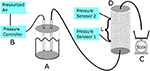Figure 2. Scheme of the Darcy column experimental setup: constant flow is imposed from the Boyle-Mariotte bottle (A) by compressed air from pressure controller (B). We measured the outflow on a scale (C) and the pressure drop at the column with two sensors (D), where the distance between the sensors defines L.

FIGURE 3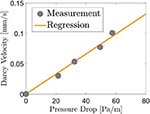Figure 3. Darcy velocity q vs. pressure drop Δp for the Newtonian fluid (66.7 vol% Glycerol + 33.3 vol% D.I. water) used in the permeability estimation, $\kappa =K{\mu }_{Newt}=4.4·1{0}^{-8}{m}^{2}$.

As a non-Newtonian fluid, we used a solution of 0.05 wt% xanthan gum produced by Sigma Aldrich. The rheology curve of the xanthan gum solution was measured with an Anton Paar MCR 702 rheometer with a double gap DG 26.7 geometry. Figure 4 shows the dynamic viscosity of our solution as a function of the applied shear rate together with the fitted Carreau model with parameters μ0 = 0.085 Pa · s, μ = 0.001 Pa · s, λ = 2 s, n = 0.48 and α = 0.8 (Equation 4). Very high shear rates have not been measured, but literature values  indicate that the viscosity of xanthan gum solutions approach the viscosity of water at high shear rates.

FIGURE 4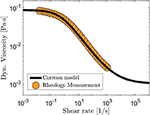Figure 4. Measured rheology of a 0.05 wt% xanthan gum solution, with the best fit of the Carreau model for the parameters: μ0 = 0.085 Pa · s, μ = 0.001 Pa · s, λ = 2 s, n = 0.48 and α = 0.8. Very high shear rates have not been measured. Literature values indicate that μ for xanthan gum solutions approximates water, i.e., μ = 0.001 Pa s .

After characterizing the non-Newtonian fluid's constitutive behavior, we perform the same Darcy experiment for the non-Newtonian solution as in the Newtonian case, measuring the pressure drop at 8 different flow rates. The effective viscosity μeff of the non-Newtonian fluid was then computed from the measured Darcy velocity q, the measured pressure drop Δp/L and the previously measured permeability κ according to

## 3. Results and Discussion

Before discussing the upscaling of our capillary model and the averaging of the viscosity approach we analyze how the profiles of velocity, shear and ultimately viscosity behave inside a single capillary. Figure 5 shows the velocity, the shear rate and the viscosity profiles, normalized by the respective means, for a capillary of radius 1 mm for two flow rates qprofile = 0.003 mm/s and qprofile = 3.7 mm/s. For the rheology of the fluid we use the Careau model with parameters μ0 = 0.085 Pa · s, μ = 0.001 Pa · s, λ = 2 s, n = 0.48 and α = 0.8. The profiles have been calculated numerically by solving Equations (22) and (27) to determine pref and ${\stackrel{˙}{\gamma }}_{w}$ together with Equations (21) and (26) as described above.

FIGURE 5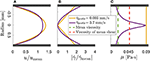Figure 5. (A) Normalized flow and (B) shear profile for flow in a capillary with an exemplary radius of 1 mm. The fluid is described by the Carreau model using the fitting parameters for the measured rheology. (C) Viscosity distribution $\mu \left(\stackrel{˙}{\gamma }\left(r\right)\right)$, including the viscosity of the mean shear rate and the mean viscosity for a mean velocity of qprofile = 3.7 mm/s.

For the low flow rate qprofile = 0.003 mm/s, the shear rate does not exceed the critical shear rate ${\stackrel{˙}{\gamma }}_{\text{crit}}=1/\lambda$ at any radial position in the capillary. Consequently the viscosity is almost constant at μ0 resulting in a Newtonian flow behavior and a parabolic velocity profile. Here the shear rate increases linearly from $\stackrel{˙}{\gamma }=0$ at the center of the capillary to $\stackrel{˙}{\gamma }={\stackrel{˙}{\gamma }}_{w}$ at the wall. As soon as the flow rate exceeds a certain threshold, nonlinear effects become important and the velocity profile flattens at the center (c.f. purple line in Figure 5A). This nonlinear behavior can also be observed in the shear rates $\stackrel{˙}{\gamma }=\text{d}u/\text{d}r$, as well as in the local viscosity μ(r) which starts to develop a very distinct maximum at the center of the capillary, Figure 5C. As a result, first averaging the shear rate and then calculating the viscosity leads to a significantly different results than calculating the average viscosity itself, namely $\mu \left(〈\stackrel{˙}{\gamma }〉\right)\ne 〈\mu \left(\stackrel{˙}{\gamma }\right)〉$. The models of Cannella et al. and Hirasaki & Pope assume a pure power-law rheology for the calculations of the effective shear rate ${\stackrel{˙}{\gamma }}_{\text{eff}}$ at all flow rates (Equations 7, 11). This does not agree with the bahavior shown in Figures 5A–C, which indicates that the profile changes from a Newtonian to a nonlinear behavior depending on the applied flow rate.

After discussing the problem of averaging shear and viscosity in a single capillary, we now benchmark the average viscosity approach. We compare our model estimates with our experimental results and the predictions of the models by Cannella et al. and Hirasaki & Pope.

Figure 6 shows the measurement of the effective viscosity as a function of the Darcy velocity using Equation (30) (red diamonds). The predictions of the models by Cannella et al. (C = 6) and Hirasaki & Pope (C = 0.69) are marked in orange and green, respectively. The solid violet line represents the prediction of our average viscosity model using a pore radius R = 0.62 mm. The characteristic pore radius R used in Figure 6 has been calculated from the bead diameter as the maximal radius of the void space between three beads that are in contact with each other (see Figure 7). Consequently we find for a bead diameter of d = 8 mm a capillary radius of R = 0.62 mm.

FIGURE 6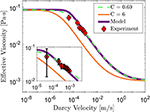Figure 6. Comparison of the experimental measurements (red diamonds) with the proposed model (purple solid line). The model is calculated for a pipe of radius R = 0.62 mm. Additionally, the correlations of Cannella et al.  (C = 6) and Hirasaki and Pope  (C = 0.69) are shown. The latter was defined from packed beds of monodisperse spheres.

FIGURE 7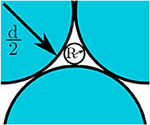Figure 7. Characteristic pore size with radius R between three glass beads with radius d/2 (blue circles).

While Cannella et al.'s model does not capture the experimental measurements, the model of Hirasaki & Pope fits the experimental data equally well as the average viscosity model. Nevertheless, all three models agree in terms of slope in the shear thinning power-law regime. This is consistent with the observation of Teeuw & Hesselink , who found that the power-law exponent of the microscopic rheology equals the exponent of the effective viscosity μeff as function of the Darcy velocity q . The major difference in the behavior of our model compared to the approach of Hirasaki & Pope  is found at the transition from the low shear Newtonian regime to the power-law regime. Here the former model predicts a slightly smoother transition than the average viscosity formulation. However, the error bars shown in the inset of Figure 6 reveal that this region also has the highest uncertainty due to the noise in the pressure measurements. Consequently, we cannot conclude that the proposed model, using an average viscosity, captures the transition between the low shear Newtonian regime and the power-law regime better than the previous models. Nevertheless, in favor of the proposed model, the linear relation in Equation (13) assumed for ${\stackrel{˙}{\gamma }}_{\text{eff}}~q$ used by both Cannella et al. and Hirasaki & Pope does not reflect the non-linear behavior of the profiles shown in Figure 5 for a single capillary.

A major challenge of models based on effective shear rates is the need of an empirical factor to compute ${\stackrel{˙}{\gamma }}_{\text{eff}}$. This, so-called C-factor, can vary over several orders of magnitude [25, 26]. This problem is especially evident considering the fact that the shape factor varies for different fluids and pore geometries . While the shape-factor C is used to fit the onset of the power-law regime in μeff against Darcy velocity plot, our model describes this transition intrinsically through the Carreau parameter 1/λ.

Comparing the models of Cannella et al. and Hirasaki & Pope reveals that the empirical shape factor C of Cannella et al.'s model corresponds to $C=1/\sqrt{\zeta }$ in the model of Hirasaki & Pope. Using the tortuosity of ζ = 25/12 for a packed bed of uniform spheres [32, 33] Hirasaki & Pope arrive at a shape factor of C = 0.69. However, Cannella et al. later found empirically that this tortuosity dependence does not hold in many different settings and proposed C = 6 as a generally good value. However if we calculate the tortuosity associated with this empirical value we obtain a tortuosity ζ = 0.03, which is inconsistent with the geometric interpretation of ζ as the elongation of flow paths in a capillary bundle model. Another problematic point of the models of Hirasaki & Pope and Cannella et al. is that the tortuosity should not matter at all for the determination of the effective viscosity as the viscosity profile does not change along the capillary. Hence the viscosity, respectively the effective shear rate, should not change with the elongation of the flow paths contrary to Equation (13).

In contrast to most currently used capillary bundle models which try to mimic the flow paths inside porous media, our average viscosity approach uses a capillary only to calculate a fully developed flow profile in a single pore. Generally, we find good agreement with experimental measurements and with previous models, provided that the free parameters have been determined from experimental data [16, 19, 32, 33, 3739]. Averaging the viscosity over the mean flow profile has several advantages. First of all, the model only includes the physical rheology of the fluid and does not need a singular power-law model as an intermediate step to calculate an effective shear rate ${\stackrel{˙}{\gamma }}_{\text{eff}}$. This eliminates the problem that arises from $\mu \left(〈\stackrel{˙}{\gamma }〉\right)\ne 〈\mu \left(\stackrel{˙}{\gamma }\right)〉$. Using the mean viscosity to describe the flow resistance of the fluid is physically more intuitive than using an effective quantity derived from comparisons of non-Newtonian flows with Newtonian behavior. Additionally our model only requires a characteristic geometric length factor rather than an empirical shape factor which depends on geometric properties as well as on the fluid. Our characteristic length scale is a property of the porous medium alone and can be estimated independently from permeability, porosity or tortuosity using e.g., pore or grain size distributions. Furthermore, our approach includes the parameter λ, which describes the onset of the shear thinning regime and is given by the rheology. Consequently, the average viscosity approach provides a consistent nonlinear upscaling of a flow profile in a pore with mean pore size and does not require tortuosity .

## 4. Conclusions

In summary, we presented a new approach to extend Darcy's law to Carreau fluids using the mean viscosity over a representative capillary as the effective flow resistance. The major advantage of the new model is that it does not require an intermediate effective shear rate and calculates the average viscosity directly using the microscopic constitutive law of the fluid. This approach allows us to upscale the average viscosity of a single pore with a mean pore size to a Darcy scale. This procedure also avoids an empirical shape parameter, which has been replaced by a characteristic length scale derived from the physical property of the porous medium itself. Furthermore, the proposed approach does not require commonly made capillary bundle assumptions like the elongation of a capillary by using the tortuosity and it does not require the permeability  to estimate an equivalent capillary diameter. Experimental measurements for a flow through a packed bed of monodisperse beads and comparison with other non-Newtonian capillary models reveal that the average viscosity provides a robust estimate for the effective Darcy viscosity μeff.

## Author Contributions

UE designed the research, carried out the experiment, performed the calculations, and analyzed the data. HS helped with the mathematical derivations and the analysis. MF assisted with the experimental setup and PB measured the rheology. JJ-M and JA supported by discussions and consulting. UE wrote the paper with input from all co-authors. MH supervised the research.

## Conflict of Interest Statement

The authors declare that the research was conducted in the absence of any commercial or financial relationships that could be construed as a potential conflict of interest.

## Acknowledgments

We kindly acknowledge Prof. Roman Stocker, Ela Burmeister, and Dr. Eleonora Secchi from the Department of Civil, Environmental and Geomatic Engineering at ETH Zürich for providing access to laboratory equipment and material. Furthermore we acknowledge Patrick Rühs from the Department of Materials and Prof. Peter Fischer from the Department for Health Sciences and Technology at ETH Zürich for suggestions and discussions. Additionally we acknowledge Nicola Gruber for cross-checking the mathematical derivations and Marius M. Neamtu Halic from the Department of Civil, Environmental and Geomatic Engineering at ETH Zürich proofreading the manuscript. JA thanks the Brazilian agencies CNPq, CAPES and FUNCAP for financial support.

## Supplementary Material

The Supplementary Material for this article can be found online at: https://www.frontiersin.org/articles/10.3389/fphy.2019.00071/full#supplementary-material

## References

1. Khaled ARA, Vafai K. The role of porous media in modeling flow and heat transfer in biological tissues. Int J Heat Mass Tran. (2003) 46:4989–5003. doi: 10.1016/S0017-9310(03)00301-6

2. Rejniak KA, Estrella V, Chen T, Cohen AS, Lloyd M, Morse DL. The role of tumor tissue architecture in treatment penetration and efficacy: an integrative study. Front Oncol. (2013) 3:111. doi: 10.3389/fonc.2013.00111

3. Cowin SC, Cardoso L. Blood and interstitial flow in the hierarchical pore space architecture of bone tissue. J Biomech. (2015) 48:842–54. doi: 10.1016/j.jbiomech.2014.12.013

4. Mackay DM, Cherry JA. Groundwater contamination: pump-and-treat remediation. Environ Sci Technol. (1989) 23:630–6. doi: 10.1021/es00064a001

5. Valdes-Abellan J, Jiménez-Martínez J, Candela L, Jacques D, Kohfahl C, Tamoh K. Reactive transport modelling to infer changes in soil hydraulic properties induced by non-conventional water irrigation. J Hydrol. (2017) 549:114–24. doi: 10.1016/j.jhydrol.2017.03.061

6. Middleton RS, Carey JW, Currier RP, Hyman JD, Kang Q, Karra S, et al. Shale and non-aqueous fracturing fluids: Opportunities and challenges for supercritical CO2. Appl Energy. (2015) 147:500–9. doi: 10.1016/j.apenergy.2015.03.023

7. Britton MM, Sederman AJ, Taylor AF, Scott SK, Gladden LF. Magnetic resonance imaging of flow-distributed oscillations. J Phys Chem A. (2005) 109:8306–13. doi: 10.1021/jp053063i

8. Wakao N, Kagei S. Heat and Mass Transfer in Packed Beds. Vol. 1. New York, NY: Taylor & Francis (1982).

9. Jackson R. Transport in Porous Catalysts. New York, NY: Elsevier Scientific Pub. Co., distributors for the U.S. and Canada, Elsevier North-Holland Amsterdam (1977).

10. Whitaker S. Flow in porous media I: a theoretical derivation of Darcy's law. Transport Porous Med. (1986) 1:3–25. doi: 10.1007/BF01036523

11. Tagliavini G, Solari F, Montanari R. CFD Simulation of a co-rotating twin-screw extruder: validation of a rheological model for a starch-based dough for snack food. Int J Food Eng. (2018) 14:32–8. doi: 10.1515/ijfe-2017-0116

12. Fischer P, Pollard M, Erni P, Marti I, Padar S. Rheological approaches to food systems. C R Phys. (2009) 10:740–50. doi: 10.1016/j.crhy.2009.10.016

13. Fischer P, Windhab EJ. Rheology of food materials. Curr Opin Colloid Interface Sci. (2011) 16:36–40. doi: 10.1016/j.cocis.2010.07.003

14. Sandvik EI, Maerker JM. Chapter 19. Application of xanthan gum for enhanced oil recovery. In: ACS Symposium Series, Vol. 45, Houston, TX: Exxon Production Research Co (1977). p. 242–264. doi: 10.1021/bk-1977-0045.ch019

CrossRef Full Text

15. López OV, Castillo LA, Ninago MD, Ciolino AE, Villar MA. Modified starches used as additives in enhanced oil recovery (EOR). In: Goyanes S, D'Accorso N, editors. Industrial Applications of Renewable Biomass Products. Cham: Springer (2017). p. 227–48.

16. Sorbie KS. Polymer-Improved Oil Recovery. New York, NY: Springer Science & Business Media (2013).

17. Sadowski TJ, Bird RB. Non-newtonian flow through porous media. I. theoretical. Trans Soc Rheol. (1965) 9:243–50. doi: 10.1122/1.549000

18. Nguyen Q, Nguyen N. Incompressible non-Newtonian fluid flows. In: Continuum Mechanics-Progress in Fundamentals and Engineering Applications. Rijeka: InTech (2012).

19. Cannella WJ, Huh C, Seright RS. Prediction of xanthan rheology in porous media. In: SPE Annual Technical Conference and Exhibition. Houston, TX: Society of Petroleum Engineers (1988).

20. Hirasaki GJPope GA, et al. Analysis of factors influencing mobility and adsorption in the flow of polymer solution through porous media. Soc Petrol Eng. (1974) 14:337–46. doi: 10.2118/4026-PA

21. Christopher RH, Middleman S. Power-law flow through a packed tube. Ind Eng Chem Res. (1965) 4:422–6. doi: 10.1021/i160016a011

22. Teeuw D, Hesselink FT. Power-law flow and hydrodynamic behaviour of biopolymer solutions in porous media. In: SPE Oilfield and Geothermal Chemistry Symposium. Stanford, TX: Society of Petroleum Engineers (1980).

23. Delshad M, Kim DH, Magbagbeola OA, Huh C, Pope GA, Tarahhom F. Mechanistic Interpretation and Utilization of Viscoelastic Behavior of Polymer Solutions for Improved Polymer-Flood Efficiency. In: SPE Symposium on Improved Oil Recovery, 20-23 April, Tulsa, OK (2008).

24. Skauge A, Zamani N, Gausdal Jacobsen J, Shaker Shiran B, Al-Shakry B, Skauge T. Polymer flow in porous media: relevance to enhanced oil recovery. Colloids Inter. (2018) 2:27. doi: 10.3390/colloids2030027

25. Wreath D, Pope GA, Sepehrnoori K. Dependence of polymer apparent viscosity on the permeable media and flow conditions. In Situ. (1990) 1:263–84.

26. Berg S, van Wunnik J. Shear Rate Determination from Pore-Scale Flow Fields. Transport Porous Med. (2017) 117:229–46. doi: 10.1007/s11242-017-0830-3

27. Carreau PJ. Rheological equations from molecular network theories. Trans Soc Rheol. (1972) 16:99–127. doi: 10.1122/1.549276

28. Bird RB. Useful non-Newtonian models. Annu Rev Fluid Mech. (1976) 8:13–4. doi: 10.1146/annurev.fl.08.010176.000305

29. Batchelor GK. An Introduction to Fluid Dynamics. Cambridge: Cambridge University Press (2000).

30. Lenk R. The Hagen-Poiseuille equation and the Rabinowitsch correction. The pressure drop in tapered channels. In: Polymer Rheology. Dordrecht: Springer (1978). p. 75–85.

31. Savins JG. Non-Newtonian flow through porous media. Ind Eng Chem. (1969) 61:18–47. doi: 10.1021/ie50718a005

32. Willhite GP, Uhl JT. Correlation of the flow of Flocon 4800 biopolymer with polymer concentration and rock properties in berea sandstone. In: Water-Soluble Polymers for Petroleum Recovery. Kansas: Springer (1988). p. 101–19.

33. Chauveteau G. Fundamental criteria in polymer flow through porous-media-and their importance in the performance differences of mobility-control buffers. Adv Chem Ser. (1986) 213:227–67. doi: 10.1021/ba-1986-0213.ch014

CrossRef Full Text

34. Abramowitz M, Stegun IA. Handbook of Mathematical Functions: With Formulas, Graphs, and Mathematical Tables. Vol. 55. Washington, DC: Courier Corporation (1965).

35. Volk A, Kähler CJ. Density model for aqueous glycerol solutions. Exp Fluids. (2018) 59:75. doi: 10.1007/s00348-018-2527-y

36. Sworn G. 8-Xanthan gum. In: Phillips GO, Williams PA, editors. Handbook of Hydrocolloids. 2nd ed. Woodhead Publishing Series in Food Science, Technology and Nutrition. Cambridge: Woodhead Publishing (2009). p. 186–203.

37. Chauveteau G. Rodlike polymer solution flow through fine pores: influence of pore size on rheological behavior. J Rheol. (1982) 26:111–42. doi: 10.1122/1.549660

38. Chauveteau G, Zaitoun A. Basic rheological behavior of xanthan polysaccharide solutions in porous media: effects of pore size and polymer concentration. In: Proceedings of the First European Symposium on Enhanced Oil Recovery, Bournemouth, England, Society of Petroleum Engineers, Richardson, TX (1981). p. 197–12.

39. Sun Y, Saleh L, Bai B. Measurement and impact factors of polymer rheology in porous media. In: Rheology. Missouri: InTech (2012).

40. Dvorkin J. Kozeny-Carman Equation Revisited. (2009). Available online at: pangea.stanford.edu/~jack/KC_2009_JD.pdf

Keywords: non-newtonian fluids, porous media, flow profile, shear rate, effective viscosity

Citation: Eberhard U, Seybold HJ, Floriancic M, Bertsch P, Jiménez-Martínez J, Andrade JS Jr and Holzner M (2019) Determination of the Effective Viscosity of Non-newtonian Fluids Flowing Through Porous Media. Front. Phys. 7:71. doi: 10.3389/fphy.2019.00071

Received: 20 December 2018; Accepted: 23 April 2019;
Published: 30 May 2019.

Edited by:

Alex Hansen, Norwegian University of Science and Technology, Norway

Reviewed by:

Laurent Talon, UMR7608 Fluides, Automatique et Systèmes Thermiques (FAST), France
Santanu Sinha, Beijing Computational Science Research Center, China

Copyright © 2019 Eberhard, Seybold, Floriancic, Bertsch, Jiménez-Martínez, Andrade and Holzner. This is an open-access article distributed under the terms of the Creative Commons Attribution License (CC BY). The use, distribution or reproduction in other forums is permitted, provided the original author(s) and the copyright owner(s) are credited and that the original publication in this journal is cited, in accordance with accepted academic practice. No use, distribution or reproduction is permitted which does not comply with these terms.

*Correspondence: Ursin Eberhard, ursine@student.ethz.ch